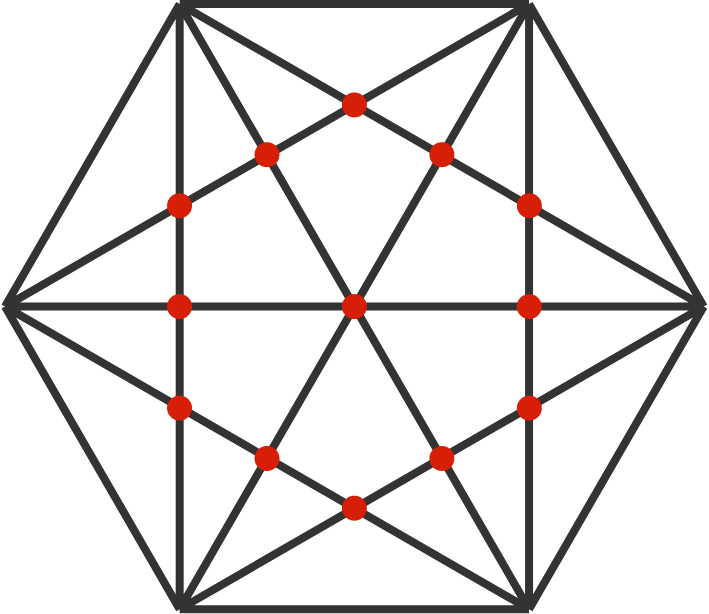# This year is always better than last year?

Probability Level 5$f(n)$ is the number of intersections of diagonals inside a regular $n$-sided polygon.

For example, in the diagram, $f(6)=13.$

Which is larger, $f(2017)$ or $f(2018)?$

×